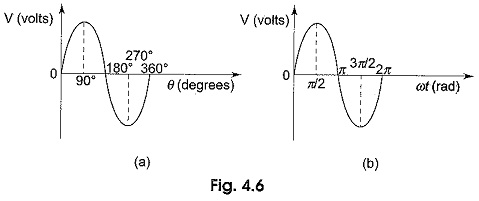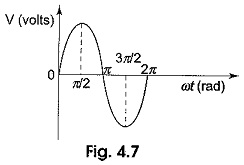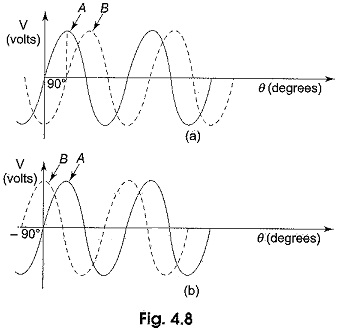## Phase of Sine Wave:

Phase of Sine Wave – A sine wave can be measured along the X-axis on a time base which is frequency-dependent. A sine wave can also be expressed in terms of an angular measurement. This angular measurement is expressed in degrees or radians.

A radian is defined as the angular distance measured along the circumference of a circle which is equal to the radius of the circle. One radian is equal to 57.3°. In a 360° revolution, there are 2π radians. The angular measurement of a sine wave is based on 360° or 2π radians for a complete cycle as shown in Figs. 4.6 (a) and (b).A sine wave completes a half cycle in 180° or π radians; a quarter cycle in 90° or π/2 radians, and so on.

The Phase of Sine Wave is an angular measurement that specifies the position of the sine wave relative to a reference. The wave shown in Fig. 4.7 is taken as the reference wave.When the sine wave is shifted left or right with reference to the wave shown in Fig. 4.7, there occurs a phase shift. Figure 4.8 shows the phase shifts of a sine wave.In Fig. 4.8(a), the sine wave is shifted to the right by 90° (π/2 rad) shown by the dotted lines. There is a phase angle of 90° between A and B. Here the waveform B is lagging behind waveform A by 90°. In other words, the sine wave A is leading the waveform B by 90°. In Fig. 4.8(b) the sine wave A is lagging behind the waveform B by 90°. In both cases, the phase difference is 90°.

Scroll to Top